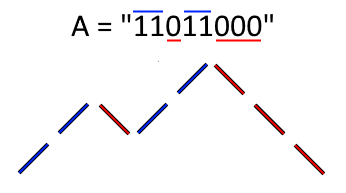## 761. Special Binary String

Special binary strings are binary strings with the following two properties:

• The number of 0's is equal to the number of 1's.
• Every prefix of the binary string has at least as many 1's as 0's.
• Given a special string S, a move consists of choosing two consecutive, non-empty, special substrings of S, and swapping them. (Two strings are consecutive if the last character of the first string is exactly one index before the first character of the second string.)

At the end of any number of moves, what is the lexicographically largest resulting string possible?

Example 1:

Input: S = "11011000"
Output: "11100100"
Explanation:
The strings "10" [occuring at S] and "1100" [at S] are swapped.
This is the lexicographically largest string possible after some number of swaps.


Note:

1. S has length at most 50.
2. S is guaranteed to be a special binary string as defined above.

b'
\n\n

#### Approach #1: Recursion [Accepted]

\n

Intuition

\n

We can represent binary strings as "up and down" drawings, as follows:

\n\n

In such a drawing, "1" is a line up one unit, and "0" is a line down one unit, where all lines are 45 degrees from horizontal.

\n

Then, a binary string is special if and only if its up and down drawing does not cross below the starting horizontal line.

\n

Now, say a special binary string is a mountain if it has no special proper prefix. When viewed through the lens of up and down drawings, a special binary string is a mountain if it touches the starting horizontal line only at the very beginning and the very end of the drawing. Notice that every special string can be written as consecutive mountains.

\n

Without loss of generality, we can assume we only swap mountains. Because if we swap special adjacent substrings A and B, and A has mountain decomposition , then we could instead swap and , then and , and so on.

\n

Also, if has mountain decomposition , and we choose to start not at the start of some , then has global height , and so cannot be special if it includes parts of another mountain as the end of mountain will cause it to dip to global height .

\n

Algorithm

\n

Say F(String S) is the desired makeLargestSpecial function. If S has mountain decomposition , the answer is , as swaps A, B involving multiple cannot have A or B start differently from where these start.

\n

It remains to determine how to handle the case when is a mountain. In that case, it must start with "1" and end with "0", so the answer is "1" + F([S, S, ..., S[N-2]]) + "0".

\n\n

Complexity Analysis

\n
\n
• \n

Time Complexity: , where is the length of .

\n
• \n
• \n

Space Complexity: .

\n
• \n
\n
\n

Analysis written by: @awice.

\n
'